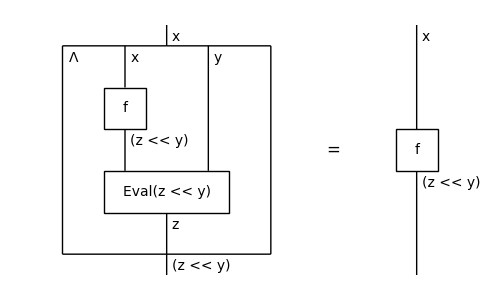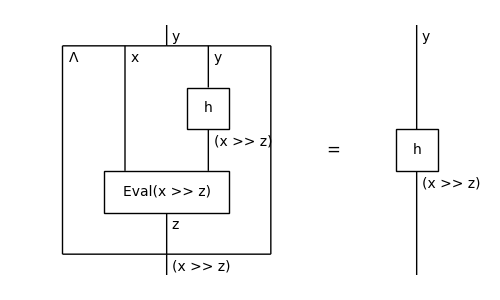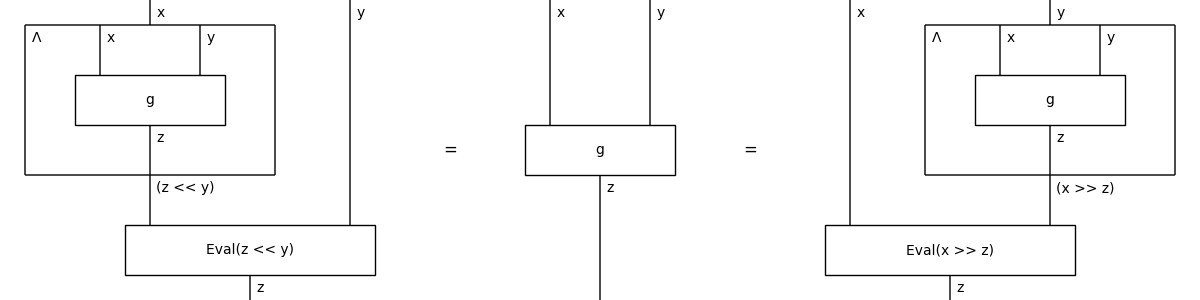# closed#

The free closed monoidal category, i.e. with exponential objects.

## Summary#

 `Ty` A closed type is a monoidal type that can be exponentiated. `Exp` A `base` type to an `exponent` type, called with `**`. `Over` An `exponent` type over a `base` type, called with `<<`. `Under` A `base` type under an `exponent` type, called with `>>`. `Diagram` A closed diagram is a monoidal diagram with `Curry` and `Eval` boxes. `Box` A closed box is a monoidal box in a closed diagram. `Eval` The evaluation of an exponential type. `Curry` The currying of a closed diagram. `Sum` A closed sum is a monoidal sum and a closed box. `Category` A closed category is a monoidal category with methods `exp` (`over` and / or `under`), `ev` and `curry`. `Functor` A closed functor is a monoidal functor that preserves evaluation and currying.

## Axioms#

`Diagram.curry()` and `Diagram.uncurry()` are inverses.

```>>> x, y, z = map(Ty, "xyz")
>>> f, g, h = Box('f', x, z << y), Box('g', x @ y, z), Box('h', y, x >> z)
```
```>>> from discopy.drawing import Equation
>>> Equation(f.uncurry().curry(), f).draw(
...     path='docs/_static/closed/curry-left.png', margins=(0.1, 0.05))
``````>>> Equation(h.uncurry(left=False).curry(left=False), h).draw(
...     path='docs/_static/closed/curry-right.png', margins=(0.1, 0.05))
``````>>> Equation(
...     g.curry().uncurry(), g, g.curry(left=False).uncurry(left=False)).draw(
...         path='docs/_static/closed/uncurry.png')
```